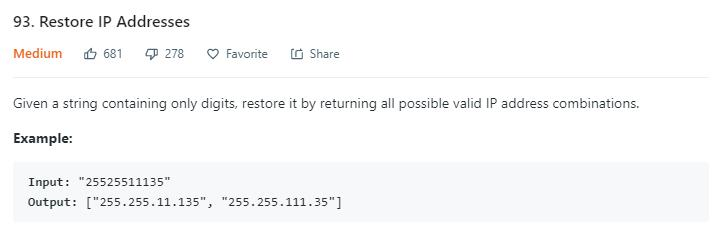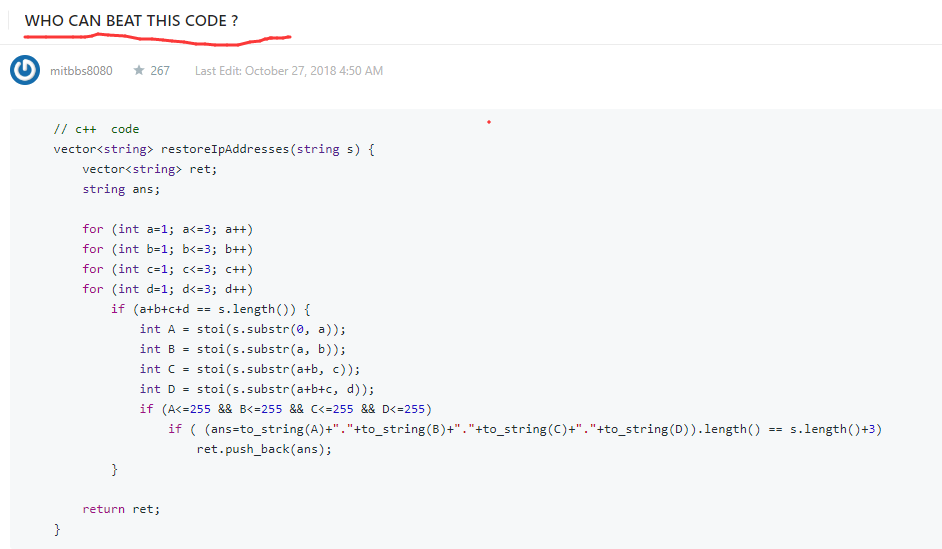# 题目描述（中等难度）# 解法一 回溯 递归 DFS

public List<String> restoreIpAddresses(String s) {
List<String> ans = new ArrayList<>(); //保存最终的所有结果
getAns(s, 0, new StringBuilder(), ans, 0);
return ans;
}

/**
* @param:  start 字符串开始部分
* @param:  temp 已经划分的部分
* @param:  ans 保存所有的解
* @param:  count 当前已经加入了几部分
*/
private void getAns(String s, int start, StringBuilder temp, List<String> ans, int count) {
//如果剩余的长度大于剩下的部分都取 3 位数的长度，那么直接结束
//例如 s = 121231312312, length = 12
//当前 start = 1，count 等于 1
//剩余字符串长度 11，剩余部分 4 - count = 3 部分，最多 3 * 3 是 9
//所以一定是非法的，直接结束
if (s.length() - start > 3 * (4 - count)) {
return;
}
//当前刚好到达了末尾
if (start == s.length()) {
//当前刚好是 4 部分，将结果加入
if (count == 4) {
}
return;
}
//当前超过末位，或者已经到达了 4 部分结束掉
if (start > s.length() || count == 4) {
return;
}
//保存的当前的解
StringBuilder before = new StringBuilder(temp);

//加入 1 位数
temp.append(s.charAt(start) + "" + '.');
getAns(s, start + 1, temp, ans, count + 1);

//如果开头是 0，直接结束
if (s.charAt(start) == '0')
return;

//加入 2 位数
if (start + 1 < s.length()) {
temp = new StringBuilder(before);//恢复为之前的解
temp.append(s.substring(start, start + 2) + "" + '.');
getAns(s, start + 2, temp, ans, count + 1);
}

//加入 3 位数
if (start + 2 < s.length()) {
temp = new StringBuilder(before);
int num = Integer.parseInt(s.substring(start, start + 3));
if (num >= 0 && num <= 255) {
temp.append(s.substring(start, start + 3) + "" + '.');
getAns(s, start + 3, temp, ans, count + 1);
}
}

}


# 解法二 迭代

public List<String> restoreIpAddresses(String s) {
List<String> res = new ArrayList<String>();
int len = s.length();
//i < 4 保证第一部分不超过 3 位数
//i < len - 2 保证剩余的字符串还能分成 3 部分
for(int i = 1; i<4 && i<len-2; i++){
for(int j = i+1; j<i+4 && j<len-1; j++){
for(int k = j+1; k<j+4 && k<len; k++){
//保存四部分的字符串
String s1 = s.substring(0,i), s2 = s.substring(i,j), s3 = s.substring(j,k), s4 = s.substring(k,len);
//判断是否合法
if(isValid(s1) && isValid(s2) && isValid(s3) && isValid(s4)){
}
}
}
}
return res;
}
public boolean isValid(String s){
if(s.length()>3 || s.length()==0 || (s.charAt(0)=='0' && s.length()>1) || Integer.parseInt(s)>255)
return false;
return true;
}


# 总